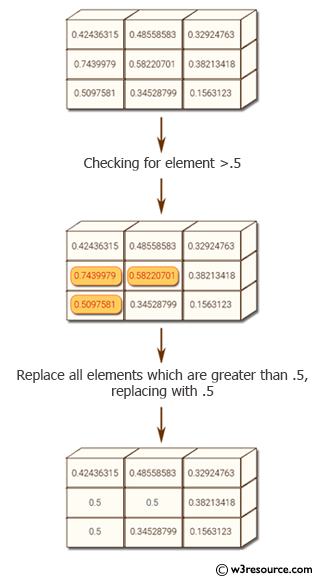﻿ NumPy: Replace all elements of numpy array that are greater than specified array - w3resource# NumPy: Replace all elements of NumPy array that are greater than specified array

## NumPy: Array Object Exercise-88 with Solution

Write a NumPy program to replace all elements of NumPy array that are greater than specified array.

Pictorial Presentation:Sample Solution:

Python Code:

``````import numpy as np
x = np.array([[ 0.42436315, 0.48558583, 0.32924763], [ 0.7439979,0.58220701,0.38213418], [ 0.5097581,0.34528799,0.1563123 ]])
print("Original array:")
print(x)
print("Replace all elements of the said array with .5 which are greater than .5")
x[x > .5] = .5
print(x)
```
```

Sample Output:

```Original array:
[[ 0.42436315  0.48558583  0.32924763]
[ 0.7439979   0.58220701  0.38213418]
[ 0.5097581   0.34528799  0.1563123 ]]
Replace all elements of the said array with .5 which are greater than .
5
[[ 0.42436315  0.48558583  0.32924763]
[ 0.5         0.5         0.38213418]
[ 0.5         0.34528799  0.1563123 ]]
```

Python Code Editor:

Have another way to solve this solution? Contribute your code (and comments) through Disqus.

What is the difficulty level of this exercise?

Test your Python skills with w3resource's quiz

﻿

## Python: Tips of the Day

List comprehension:

```>>> m = [x ** 2 for x in range(5)]
>>> m
[0, 1, 4, 9, 16]
```